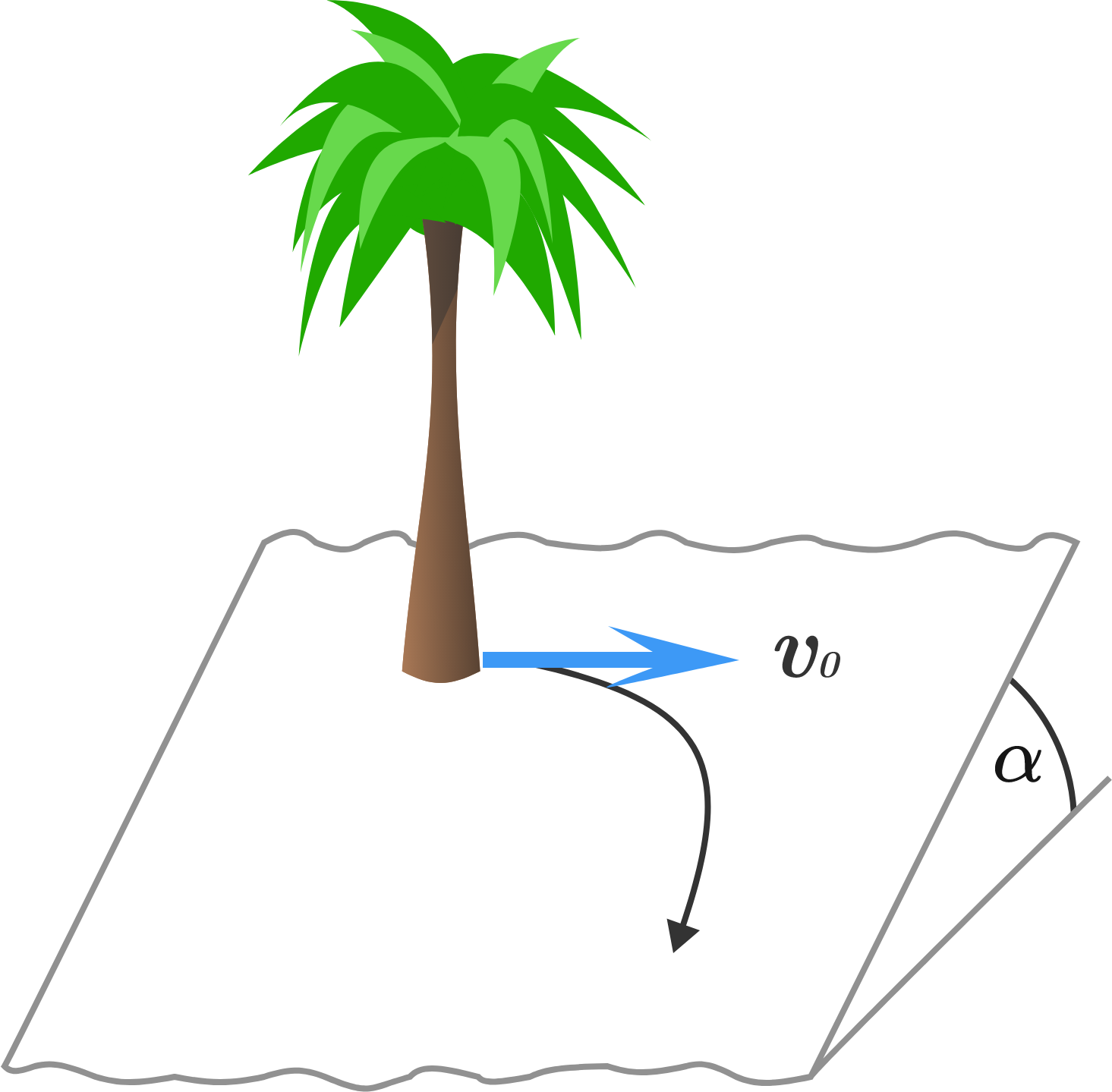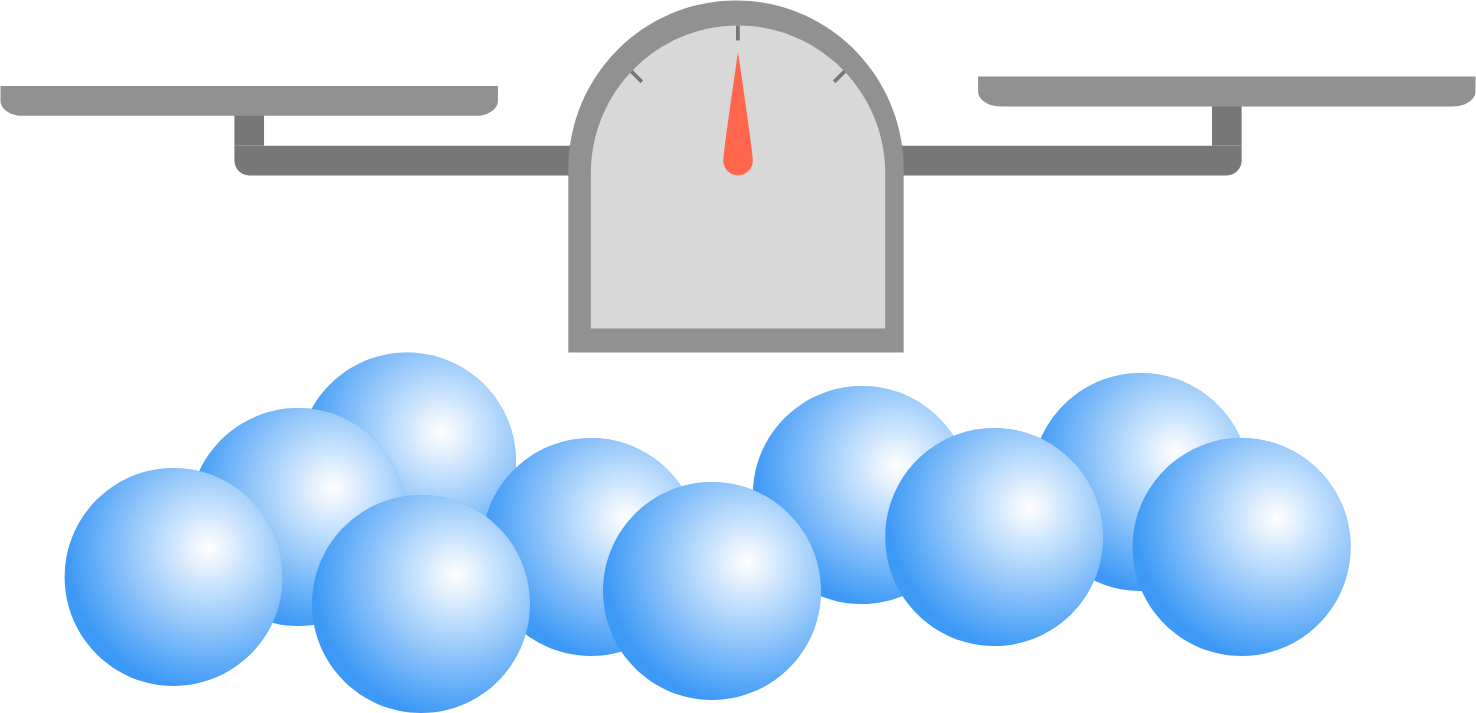# 2017-02-27 Advanced

$\left \lfloor \frac{2}{1}\times\frac{5}{4}\times\frac{8}{7}\times\frac{11}{10}\times\cdots\times\frac{2015}{2014}\times\frac{2018}{2017} \right \rfloor = \, ?$

Notation: $\lfloor \cdot \rfloor$ denotes the floor function.

Two children stand on a large sloping hillside that can be considered as a plane. The angle of the incline of the hillside is $\alpha$. The ground is just sufficiently icy that a child would slide downhill with uniform speed as a result of receiving even the slightest impulse down the hill.

For fun, one of the children (leaning against a tree) pushes the other child with a horizontal initial speed of $v_0 = \SI{1}{m/s}$, who then slides down the slope, as indicated by the curved arrow. What will be this child's final speed (in $\si{m/s}$) if air drag is negligible and the frictional force is independent of speed?Suppose there are 10 balls identical in appearance, where 8 of them each have a mass of $x$ grams, and each of the other two $(x + \delta)$ grams. Now, $\delta \, (\gt 0)$ is so small that the difference can't be detected using your own hands, and can only be detected by the precise balance scale you have been provided with. You can put any number of balls in each pan of the scale.

What is the minimum number of weighings you will need to make to guarantee the identification of both of the heavier balls?$\large r = \lim_{n\to\infty} \sqrt[n]{1 + \dfrac{n^2}{(n+1)^2}} \cdot \sqrt[n]{1 + \dfrac{n^2}{(n+2)^2}} \cdots \sqrt[n]{1 + \dfrac{n^2}{(n+n^4)^2}}$

Find the value of the closed form of $r$ to 3 decimal places.Is it possible to place (infinitely many) closed discs (of positive radius) in a unit square, where the interiors of the discs do not intersect, such that any point given in the interior of the square lies on a disc?

• The interior of each disc must be contained in the unit square. The perimeter of the disc can touch the perimeter of the square.
• The interiors of 2 discs do not intersect. The perimeters of the discs are allowed to intersect (so they touch each other).
• Note that the corners of the square cannot be covered by a closed disc, which is why I'm asking about the interior.
×

Problem Loading...

Note Loading...

Set Loading...